Courses
Courses for Kids
Free study material
Free LIVE classes
More

# NCERT Exemplar for Class 9 Science Chapter 12 - Sound## NCERT Exemplar for Class 9 Science - Sound - Free PDF Download

Last updated date: 30th May 2023
Total views: 556.8k
Views today: 10.53k

## Access NCERT Exemplar Solutions for Class 9 Science(Physics) Chapter 12 - Sound

### Multiple Choice Questions

1. Note is a sound

(a) of mixture of several frequencies

(b) of mixture of two frequencies only

(c) of a single frequency

(d) always unpleasant to listen

Ans: (a) of mixture of several frequencies

Explanation: Note is a sound of mixture of several frequencies and is pleasant of hear.

2. A key of a mechanical piano struck gently and then struck again but much harder

this time. In the second case

(a) sound will be louder but pitch will not be different

(b) sound will be louder and pitch will also be higher

(c) sound will be louder but pitch will be lower

(d) both loudness and pitch will remain unaffected

Ans: (a) sound will be louder but pitch will not be different

Explanation: The pitch depends on frequency of the particular key which is being hit and hence there would be no change in pitch of sound. Loudness depends on amplitude which will be more if the key is struck harder.

3. In SONAR, we use

(a) ultrasonic waves

(b) infrasonic waves

(d) audible sound waves

Ans: (a) ultrasonic waves

4. Sound travels in air if

(a) particles of medium travel from one place to another

(b) there is no moisture in the atmosphere

(c) disturbance moves

(d) both particles as well as disturbance travel from one place to another.

Ans: (c) disturbance moves

Explanation: Sound propagation does not need particle mobility. It merely takes the disruption to go from one particle to the next.

5. When we change feeble sound to loud sound we increase its

(a) frequency

(b) amplitude

(c) velocity

(d) wavelength

Ans: (b) amplitude

Explanation: The amplitude of a sound determines its loudness, which rises as the amplitude rises.

6. In the curve (Fig.12.1) half the wavelength is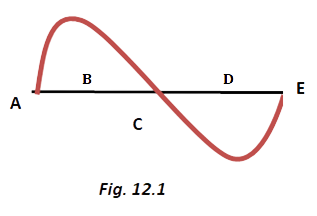(a) AB

(b) BD

(c) DE

(d) AE

Ans: (b) BD

Explanation: The wavelength is defined as the distance between two successive peaks or troughs. One peak and one dip can be seen in the graph. The sum of the peak and trough widths equals the wavelength, which in this case is AE. As a result, BD has a wavelength of half that of BD.

7. Earthquake produces which kind of sound before the main shock wave begins

(a) ultrasound

(b) infrasound

(c) audible sound

(d) none of the above

Ans: (b) infrasound

Explanation: Some animals receive prior notice of an earthquake thanks to infrasound and exhibit unusual behaviour as a result.

8. Infrasound can be heard by

(a) dog

(b) bat

(c) rhinoceros

(d) human beings

Ans: (c) rhinoceros

Explanation: Rhinoceros communicate by emitting infrasound at frequencies as low as 5 Hz.

9. Before playing the orchestra in a musical concert, a sitarist tries to adjust the tension

and pluck the string suitably. By doing so, he is adjusting

(a) intensity of sound only

(b) amplitude of sound only

(c) frequency of the sitar string with the frequency of other musical instruments

(d) loudness of sound

Ans: (c) frequency of the sitar string with the frequency of other musical instruments

Explanation: A musical instrument's frequency should be in sync with the frequency of other musical instruments. This helps to make music that is pleasing to listen to. As a result, at the commencement of a musical performance, artists routinely change the frequencies.

10. The given graph (Fig.12.2) shows the displacement versus time relation for a

disturbance travelling with velocity of 1500 ms–1. Calculate the wavelength of the disturbance.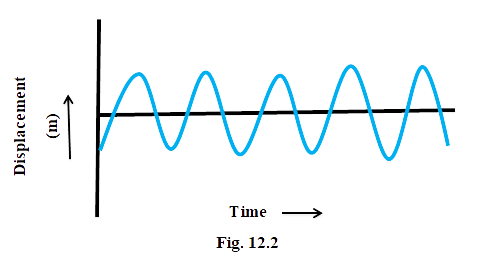Ans: From the graph

Time period, $t = 2 \times {10^{ - 6}}s$

Frequency,    $v = \frac{1}{t} = 5 \times {10^5}Hz$

Wavelength,   $\lambda = \frac{u}{v} = 5 \times {10^5}m$

11. Which of the two graphs (a) and (b) (Fig.12.3) representing the human voice is likely to be the male voice? Give reason for your answer.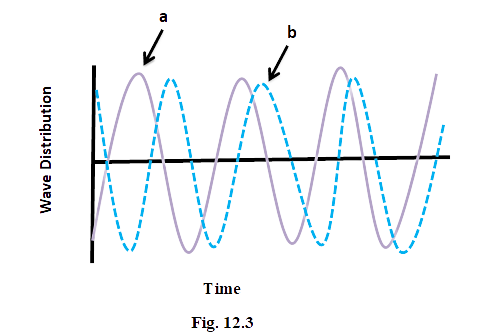Ans: Graph (a) represents the male voice. Usually the male voice has less pitch (or frequency) as compared to female.

12. A girl is sitting in the middle of a park of dimension 12 m × 12 m. On the left side of it there is a building adjoining the park and on right side of the park, there is a road adjoining the park. A sound is produced on the road by a cracker. Is it possible for the girl to hear the echo of this sound? Explain your answer.

Ans: If the time gap between the original sound and reflected sound received by the listener is around 0.1 s, only then the echo can be heard.

The minimum distance travelled by the reflected sound wave for distinctly listening to the echo.

= velocity of sound × time interval

= 344 x 0.1

= 34.4m

But in this case the distance travelled by the sound reflected from the building and then reaching to the girl will be (6 + 6) = 12 m, which is much smaller than the required distance. Therefore, no echo can be heard.

13. Why do we hear the sound produced by the humming bees while the sound of Vibrations of pendulum is not heard?

Ans: Humming bees make sound by shaking their wings, and this sound is audible (20Hz to 20.000 Hz). The frequency of a pendulum is less than 20 Hz, which is below the audible range.

14. If any explosion takes place at the bottom of a lake, what type of shock waves in water will take place?

Ans: Because sound waves are longitudinal waves, they are referred to as longitudinal waves.

15. Sound produced by a thunderstorm is heard 10 s after the lightning is seen. Calculate the approximate distance of the thunder cloud. (Given speed of sound = 340 ms–1.)

Ans: Speed of sound = 340 m/s and time = 10 s

Distance = speed × time = 340 × 10 = 3400 m

16. For hearing the loudest ticking sound heard by the ear, find the angle x in the Fig.12.4.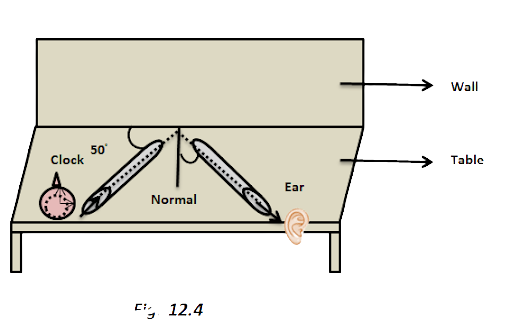Ans: Incident line is making an angle of 50owith reflecting surface.

So, angle of incidence = 90o– 50o= 40o

Angle of reflection = angle of incidence

Hence, x=40°

17. Why is the ceiling and wall behind the stage of good conference halls or concert

Ans: The ceiling and walls are curved to ensure that sound hits all audience members evenly in all directions after reflection.

18. Represent graphically by two separate diagrams in each case

(i) Two sound waves having the same amplitude but different frequencies?

Ans: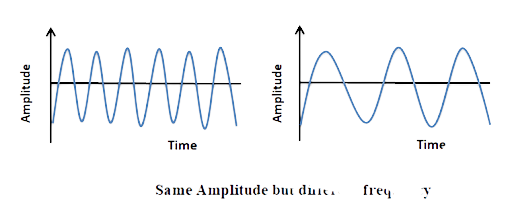(ii) Two sound waves having the same frequency but different amplitudes.

Ans: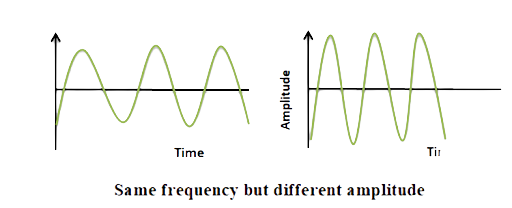(iii) Two sound waves having different amplitudes and also different wavelengths.

Ans: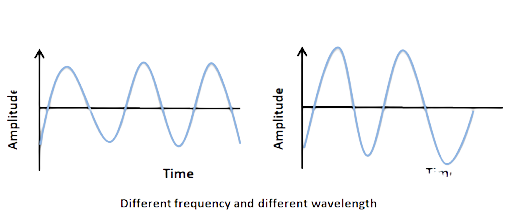19. Establish the relationship between the speed of sound, its wavelength and frequency. If the velocity of sound in air is 340 ms–1, calculate

(i) wavelength when the frequency is 256 Hz.

Ans: u= v λ

340=  256 λ

λ= 1.33m

(ii) frequency when wavelength is 0.85 m.

Ans: 340 = v (0.85)

v= 400 Hz

20. Draw a curve showing density or pressure variations with respect to distance for a disturbance produced by sound. Mark the position of compression and rarefaction on this curve. Also, define wavelengths and time-period using this curve.

Ans: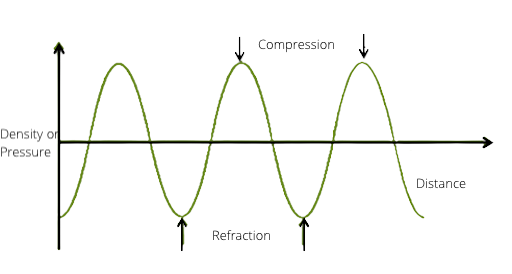The distance between two consecutive compressions or rarefactions is measured in wavelength. The time it takes to travel the distance between any two successive compressions or rarefactions from a fixed point is called the time period.

### Science NCERT Exemplar for Chapter 12 (Sound) of Class 9

Chapter 12 of Science NCERT deals with the fundamental information about sound. Various concepts are discussed in this Chapter. These concepts will help build a concrete foundation for the students that will help them in higher studies and also in qualifying competitive exams.

### Key Points in Chapter 12 of Class 9 Science NCERT

Some of the important points that are discussed in Chapter 12, that is, sound of Class 9th NCERT are:

• Vibrations of different objects produce sound.

• Sound travels in the form of longitudinal waves through a material medium.

• While travelling, sound forms successive compressions and rarefactions in the medium.

• During sound propagation, it is not the particles of the medium but the energy of the sound that travels.

• Sound travel is not possible in a vacuum.

• One complete oscillation refers to the change in density from maximum to minimum and back to maximum.

• The period in which a wave completes one oscillation is referred to as a period and is denoted with a T.

• The wavelength of sound is the distance between two consecutive compressions or rarefactions and it is denoted by lambda.

• The number of oscillations completed per unit time is referred to as frequency. It is denoted with v and its value is the inverse of the period, that is, 1/T.

• The speed with which sound travels in a medium depends on the nature and temperature of the medium in which it is travelling.

• The law of reflection of sound is an important law that explains various day-to-day phenomena like the occurrence of sound in an auditorium.

• The repeated reflections of sound are known as reverberation and usually occur in the auditorium.

• The properties of sound waves help in determining basic properties of sound like pitch, sound quality, and loudness.

• The intensity of sound is referred to as the amount of sound energy that passes each second through a unit area.

• An average human being can hear sounds that have a frequency range of 20 Hz - 20 kHz.

• Sound waves that are below 20 Hz are known as infrasonic and sound waves above 20 kHz are known as ultrasonic.

• The SONAR technique uses ultrasonic waves to predict the depth of the sea and locate submarines, sunken ships, icebergs, valleys, and water hills.

## FAQs on NCERT Exemplar for Class 9 Science Chapter 12 - Sound

1. What topics are included in Chapter 12 of the Class 9 Science Exemplar?

Chapter 12 of Class 9 Science exemplar contains questions of topics of utmost importance like:

• Sound production

• Sound propagation

• Sound waves and their characteristics

• Speed of sound in different media

• Reflection of sound and reverberation

• Echo

• Range of hearing

• Ultrasound and its applications

• Sonar

• Structure of the human ear

It is beneficial for students of Class 9 to download NCERT exemplar as this exemplar:

• Contains solutions to questions present in NCERT textbook and NCERT exemplar book.

• The solutions to each answer are provided in the simplest possible way so that it is understandable by Class 9 students.

• The solutions are strictly based on the CBSE syllabus and not concepts different from the syllabus that has been discussed.

• The questions are prepared in a pattern that is similar to the examination pattern and may help students during boards.

3. What type of questions are present in Chapter 12 of the Science NCERT exemplar?

The NCERT exemplar contains questions in the form of multiple-choice questions (MCQs), long answer questions, and short answer questions. These questions cover all levels ranging from easiest and then moving towards difficult questions. The exemplar contains 9 multiple-choice questions (MCQs), 8 short answer questions, and 3 long answer questions that cover all important concepts of Chapter 12 of Class 9 Science. These questions cover the complete syllabus of Science Chapter 12 prescribed in NCERT textbooks for Class 9. Thus, it will be beneficial for the students to go through all these questions.

4. How can Vedantu help me in preparing for my exams?

Vedantu contains a team of subject experts that prepare solutions for important questions from an examination point of view. These solutions are free to download and are available on the official website of Vedantu. Vedantu not only gives solutions to the NCERT exemplar but also covers extra questions, sample papers, and important questions from the previous year's question paper. It also provides students with certain tips and tricks that can be beneficial for the students in not only Class 9 examination but also in higher Classes and competitive examinations.

5. How can I ensure that I can answer all questions of Chapter 12 (Science) that will appear in the question paper?

Here are certain tips from our experts that will surely help you in attempting questions from Chapter 12, that is, sound:

• A good understanding of the basic concepts is very important as that will only help you to solve difficult questions easily.

• Practice numerical as much as you can so that you don't make any silly mistakes while attempting your question paper.

• Practice important diagrams given in the Chapter, like, the structure of the human ear so that you can make a well-labelled diagram in your exam and don't lose any marks there.